# Introduction !!

Generative Adversarial networks(GAN’s) were introduced by Ian goodfellow in his white paper written in 2014(https://arxiv.org/abs/1406.2661). GAN’s are basically  based on two simple principles that are generative modelling and adversarial training.

# Generative Modelling !!

Basically there are two types of models :-

### -> Probabilistic Discriminative model :-

• This model takes a input and returns the probability of type of the input as its output.
• Direct modelling of the posterior probability that is probability of output(y) given the input(x) :- p(y|x)
• Main motive of this type of models is to do mapping between the inputs and outputs.
• Example :- Convolution neural networks(image classification).### -> Generative model :-

• Computation of posterior probabilities using Bayes rule : Class-conditional densities and class priors.
• Modelling is done by using joint probability of having a input(x) as well as its type (distinct feature:y) :- J(x/y)
• Main motive of such type of model is to learn to generate input type data.
• Example :- Variational auto-encoders(handwriting generation).• It simply means training a model on adversarial inputs that is our worst case inputs.
• Inputs to the model are chosen by an adversary.
• Example :- A video game bot playing with copy of itself .
• Basically it is like practicing mathematics on most difficult problems to understand the concept more clearly.• In a nutshell it can be explained as a game played between two players, Job of  one player is to fool the other player while other player tries its best to not be fooled .
• Both of these players are neural networks . One neural network is a discriminative networks of course that means its is based on probabilistic discriminative modelling. While, other network is generative network and is based on generative modelling technique.
• Now the generative neural network learns to produce the worst case inputs for discriminative neural network and discriminative neural network tries to predict correctly whether the input is a real training input or a fake input produced by the generative network.Main objective -> It is o learn to generate data that resembles the training dataset.

## Structure and Working of a GAN Model –>

• One neural network that generates called generator.
• One neural network that discriminates called discriminator.
• Goal of Generator is to fool the discriminator.
• Both Neural nets gets better at their job during the process of training.
• Finally, Generator is forced to generate a image as realistic as possible.

## Steps :-

Discriminator –>

• Let a sampled image from training data is our input x.
• Discriminator applies a differentiable function D(x),Which will try to predict whether the image is a real input or an adversarial input.
• Objective of Discriminator function D(x) is to be as close to 1 that is it predicts the input type correctly almost in every iteration.

Generator –>

• Generator add some noise to the input x .
• This noise then constitutes with a generative function G.
• This function G(z) then generates our adversarial input.

Objectives :-

• Discriminator tries to make D(G(z)) near to 0 that is it catches a fake input every time.
• While Generator tries to make G(z) =~ x , So that D(G(z)) will be equal to 1.# MINIMax Game Analogy :-

The Generator Network takes an random input and tries to generate a sample of data. In the above image, we can see that generator G(z) takes a input z from p(z), where  z is a sample from probability distribution p(z). It then generates a data which is then fed into a discriminator network D(x). The task of Discriminator Network is to take input either from the real data or from the generator and try to predict whether the input is real or generated. It takes an input x from pdata(x) where pdata(x) is our real data distribution. D(x) then solves a binary classification problem using sigmoid function giving output in the range 0 to 1.Let us define the notations we will be using to formalize our GAN,

Pdata(x) -> the distribution of real data
X -> sample from pdata(x)
P(z) -> distribution of generator
Z -> sample from p(z)
G(z) -> Generator Network
D(x) -> Discriminator Network

Now the training of GAN is done (as we saw above) as a fight between generator and discriminator. This can be represented mathematically as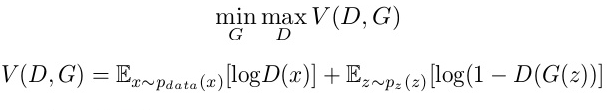In our function V(D, G) the first term is entropy that the data from real distribution (pdata(x)) passes this to 1. The second term is entropy that the data from random input (p(z)) passes through the generator, which then generates a fake sample which is then passed through the discriminator to identify the fakeness (aka worst case scenario). In this term, discriminator tries to maximize it to 0 (i.e. the log probability that the data from generated is fake is equal to 0). So overall, the discriminator is trying to maximize our function V.On the other hand, the task of generator is exactly opposite, i.e. it tries to minimize the function V so that the differentiation between real and fake data is bare minimum. This, in other words is a cat and mouse game between generator and discriminator! This method of training a GAN is taken from game theory called the minimax game.

# Understanding by a classic example:-

• Classic example that is used to explain the working of generative adversarial network is of counterfeiters and police.
• Now the job of police is to recognize counterfeit currency and job of counterfeiters is to generate as realistic counterfeit currency , such that even police is not able recognize it.
• Both try to better their jobs with training but in the end when counterfeiters are able to make exact copy of real currency, Even police Fails to recognize the difference between real and fake. (Of course, in real life only option left under such situation is demonetization.)
• So police plays the role of discriminator and counterfeiters plays the role of generator.

# Applications of GAN’s :-

Predicting the next frame in a video : GAN’s Can be trained to predict the next frame of the video by providing the previous time frames as inputs.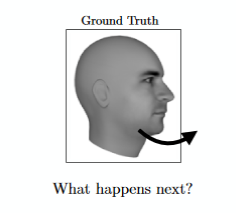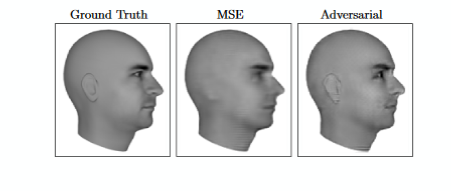Paper : https://arxiv.org/pdf/1511.06380.pdfhttps://arxiv.org/pdf/1511.06380.pdf

• Increasing Resolution of an image : Low resolution image can be converted into high resolution image.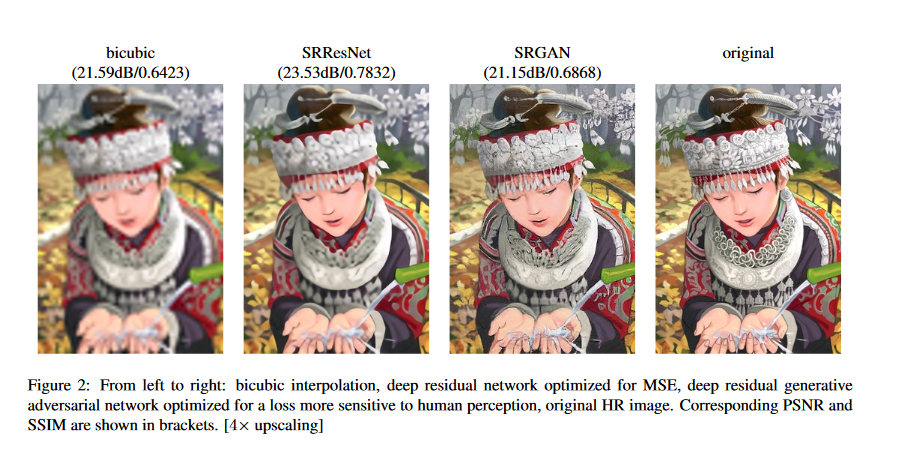Paper: https://arxiv.org/pdf/1609.04802.pdf

• Interactive Image Generation : Draw simple strokes and let the GAN draw an impressive picture for you!• Text to Image Generation : Give a description of an image and GAN will produce them for you.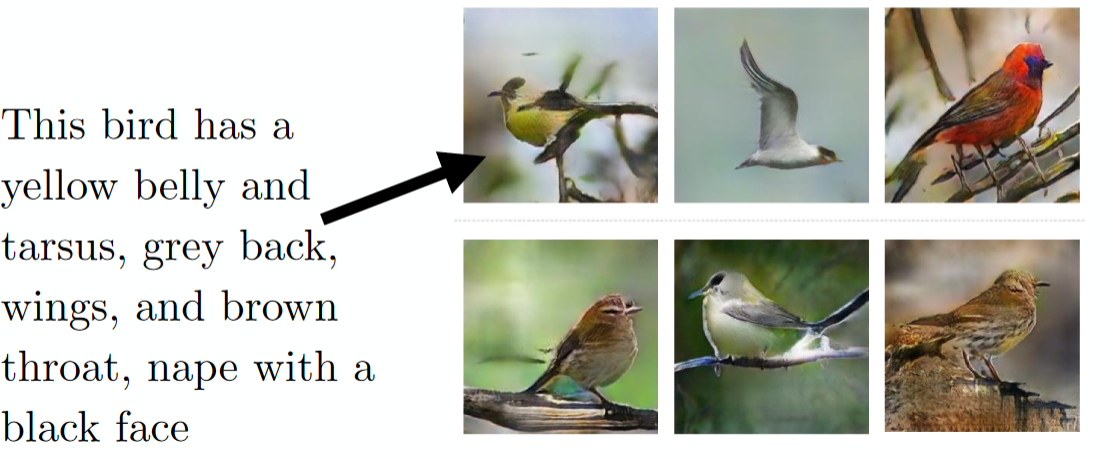Paper : https://arxiv.org/pdf/1605.05396.pdf

# Coming Next –>

• Different types of Generative adversarial networks and their working.
• Implementation of GAN using keras library.